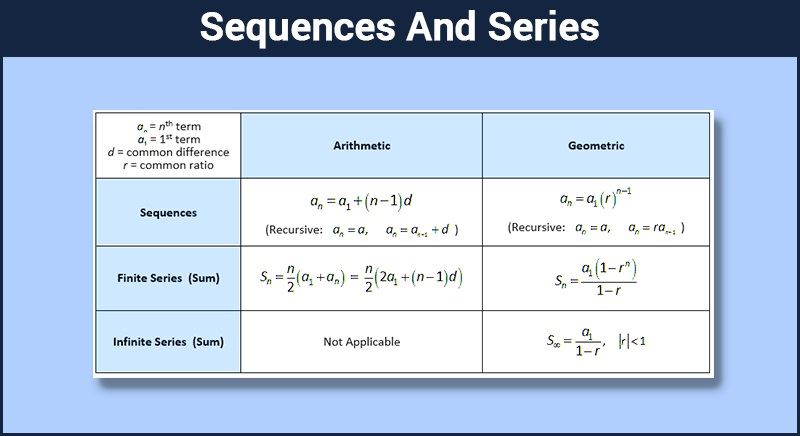# Sequences And Series

To understand sequences and series, let us start with an interesting question.

There was a pair of young rabbits. The pair of rabbits grew up during the next month. So, at the end of first and the second month, there was 1 pair of rabbits. After next month, they gave birth to another pair of rabbits. So, there were two pairs at the end of the third month. The young rabbits grew up for the next month and the old pair gave birth to a new pair. So, at the end of fourth month, there were 3 pairs. At the end of the fifth month, there were two pairs which gave birth to a pair each. Also, there was that young pair from the last month. So, the number of total pairs at the end of fifth month was 5. Assuming that no rabbit dies and runs away, how many pairs of rabbits will be there after a year?The answer to this question created something which is used by us in day to day life. If the question is analyzed something and we write it in form of a table, it would look something like this:

 No. of month(s) passed No. of pair(s) of rabbits 1 1 2 1 3 2 4 3 5 5 . . . . . . . . 12 ???

If observed carefully, a pattern can be observed in the no. of pairs of rabbits. After each month, the number is equal to the sum of the number of pairs in the previous two months. Like this, we can go ahead and write the full sequence till 12 months as

$1, 1, 2, 3, 5, 8, 13, 21, 34, 55, 89, 144$

So, we can conclude that no. of pairs of rabbits after a year would be $144$. The understanding of the pattern helped us in getting a quick answer to that problem. The person who answered that question and discovered that pattern was Fibonacci. This list of numbers, after him, is known as the Fibonacci sequence. Let us understand what a sequence is.

Definition of Sequences and Series:

Definition 1: A sequence is an ordered list of objects or numbers. The numbers or objects are also known as the terms of the sequence.

Some examples are:

• $1, 2, 3, 4, 5, …$ is the sequence of natural numbers.
• $\frac{1}{2}, \frac{1}{4}, \frac{1}{6}, \frac{1}{8},…$ is the sequence of multiplicative inverse of multiples of $2$.
• $Rs.~10000, Rs.~15000, Rs.~16000, Rs.~20000,~…$ can be the sequence of savings for consecutive months for somebody.
• $0, 1, -1, 2, -2, …$ is the sequence of integers.

A sequence can be finite or infinite depending upon number of terms in it. Let us now understand what series is. A series is derived from a sequence.

Definition 2: Series is defined as the sum of the products of a sequence.

Corresponding series for the above examples of sequence are:

• $1 + 2 + 3 + 4 + 5 + ⋯$
• $\frac{1}{2} + \frac{1}{4} + \frac{1}{6} + \frac{1}{8} + ⋯$
• $Rs.~10000 + Rs.~15000 + Rs.~16000 + Rs.~20000 + …$
• $0 + 1 – 1 + 2 -2 + ⋯$

So, that’s the difference between a sequence and s series. These terms are not interchangeable, at least not for mathematicians.

A progression is a special sequence. If it is possible to obtain a formula to represent the $n^{th}$ term of a sequence, that sequence is a progression. So, all progressions are sequences but the converse may or may not be true.

To learn more about sequences and series, Download Byju’s – The Learning App from Google Play Store and explore interactive learning through interesting videos.

#### Practise This Question

What are the conditions for a sequence to be an AP?